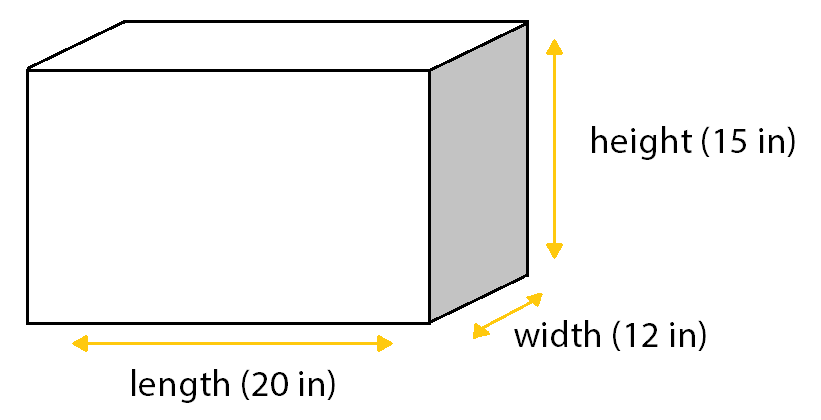# TEAS Math Practice Test

Prepare for your test with realistic questions.

The Math section of the TEAS requires you to use algebra, numbers, measurements, and data to solve problems successfully. To help you prepare for this section of the TEAS, this page contains everything you need to know, including what topics are covered, how many questions there are, and how you can study effectively.

## What’s on the TEAS Math Section?## TEAS Online Course

If you are wanting to be fully prepared, Mometrix offers an online TEAS prep course. The course is designed to provide you with any and every resource you might want while studying. The TEAS course includes:

• 106 Lessons Covering Every Topic
• Over 2,100 TEAS Practice Questions
• 200+ Video Tutorials
• 310+ Electronic Flashcards
• Money-back Guarantee
• Easy Mobile Access
• and More!

The TEAS prep course is designed to help any learner get everything they need to prepare for their TEAS exam. Click below to check it out!## TEAS Math Example Problems

Here are three example questions from the TEAS Math exam, each with a simple explanation showing how the problem is solved.

### 1. Solve for $$x$$: $$2x+4=x–6$$

To solve an equation with the variable on both sides of the equal sign, you must rearrange the terms. Specifically, use inverse operations of addition or multiplication to gather the variable terms to one side, and the constants to the other:

Step 1: Move the $$x$$-term from the right side of the equation to the left by subtracting $$x$$ from both sides of the equation.Step 2: Move the constant term, 4, from the left side to the right by subtracting 4 from both sides of the equation.Therefore, the solution is $$x=-10$$.

### 2. How many cubic inches of water could this aquarium hold if it were filled completely?

This question is asking for the volume of a rectangular aquarium with given dimensions of length, width and height. The formula to determine the volume is: $$V = l \cdot w \cdot h$$Substitute these dimensions into the formula:

$$V=(20)(12)(15)$$ $$V=3,600$$

In context, this means that the aquarium shown will hold 3,600 cubic inches of water if it were filled completely.

### 3. Solve this word problem:

A lab technician drew 400 milliliters of blood from a patient. 1/7 of the blood was used for further testing. How many milliliters of blood were used for those further tests? Round the answer to the nearest hundredth.

In real-life applications, there are often keywords that translate directly to a math operation. In this example, you need to determine the amount of blood that represents of 400 milliliters.  The word of translates to multiply.

Determine the amount of blood used for other tests by multiplying the fraction by the amount of blood drawn:

$$400 \times \frac{1}{7} \Rightarrow \frac{400 \times 1}{7} \Rightarrow \frac{400}{7} \Rightarrow 57.14285714$$ milliliters

Round this decimal to the nearest hundredth and interpret in context:

57.14 milliliters of blood were used for further testing.

## FAQs

### Who takes the TEAS?

#### A

The TEAS is designed for students seeking admission into nursing or allied health programs. Many educational institutions require students to take the TEAS. Students are encouraged to learn more about their school’s score requirements.

### How many questions are on the TEAS Math exam?

#### A

The Math section of the TEAS contains 38 questions. This section includes four “pretest” questions that do not count toward your final score.

### How long is the TEAS Math exam?

#### A

Students have 57 minutes to complete the Math section, which has 38 questions.

### Can you use a calculator for the TEAS?

#### A

Yes. On test day, a test administrator will provide you with a four-function calculator (depending on the location). Calculators with built-in or specialized functions are not allowed.

### What is a passing TEAS Math score?

#### A

The nursing program of your choice determines the score requirements for TEAS. At the end of the exam, students will receive a “main” or total score that accumulates all four sections (Science, Math, Reading, and English & Language Usage). Students will also receive separate scores for each section. Many nursing programs require students to earn a total score of at least 60% – 70%. Students are encouraged to score higher than the program’s minimum score requirement to increase their chances of admission.

by Mometrix Test Preparation | This Page Last Updated: October 29, 2023

Get 25% Off Your TEAS Prep Course
Get a discount code for our comprehensive TEAS Online Prep Course! All of our resources in one place for one low price.
Get 25% Off Your TEAS Prep Course
Get a discount code for our comprehensive TEAS Online Prep Course! All of our resources in one place for one low price.